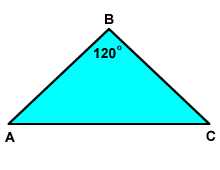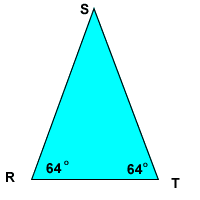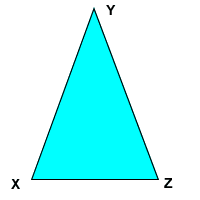# Isosceles Triangles Have Two Equal Sides

There are many types of triangles in the world of geometry. There is a special triangle called an isosceles triangle. In an isosceles triangle, the base angles have the same degree measure and are, as a result, equal (congruent). Similarly, if two angles of a triangle have equal measure, then the sides opposite those angles are the same length. The easiest way to define an isosceles triangle is that it has two equal sides.

In an isosceles triangle, we have two sides called the legs and a third side called the base. The angle located opposite the base is called the vertex.

### Sample A:

The vertex angle B of isosceles triangle ABC is 120 degrees. Find the degree measure of each base angle.Solution:

(1) Let x = the measure of each base angle.
(2) Set up an equation and solve for x.

base angle + base angle + 120 degrees = 180 degrees
x + x + 120 degrees = 180 degrees
2x + 120 = 180
2x = 180 - 120
2x = 60
x = 60/2
x = 30

Each base angle of triangle ABC measures 30 degrees.

### Sample B:

In isosceles triangle RST, angle S is the vertex angle. Base angles R and T both measure 64 degrees. Find the degree measure of the vertex angle S.Solution:

(1) Let x = measure of vertex angle S.
(2) Set up an equation and solve for x.

base angle + base angle + vertex angle S = 180 degrees
64 degrees + 64 degrees + x = 180 degrees
128 + x = 180
x = 180 - 128
x = 52

The measure of vertex angle S in triangle RST is 52 degrees.

### Sample C:

The degree measure of a base angle of isosceles triangle XYZ exceeds three times the degrees measure of the vertex Y by 60. Find the degree measure of the vertex angle Y. Notice that it's hard to draw a picture without knowing which angles are largest.We need to make an equation out of this problem, so let's figure out what it's trying to tell us. First we read "The degree measure of a base angle", so let's start with X=

Our equation so far: X=

Now we see "exceeds three times... Y... by 60", which means 3Y + 60.

Our equation now: X = 3Y + 60

Since we know that X = Z because it is an isosceles triangle, then we can solve for the measures of all the angles.

base angle + base angle + vertex = 180
X + Y + Z = 180
(3Y + 60) + Y+ (3Y + 60) = 180
7Y + 120 = 180
7Y = 60
Y = 60/7
Y = 8.57 degrees

The vertex angle Y of triangle XYZ equals 8.57 degrees.

Lesson provided by Mr. Feliz